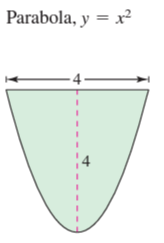×
Get Full Access to Calculus: Early Transcendental Functions - 6 Edition - Chapter 7.7 - Problem 11
Get Full Access to Calculus: Early Transcendental Functions - 6 Edition - Chapter 7.7 - Problem 11

×

# ?Fluid Force on a Tank Wall In Exercises 7-12, find the fluid force on the vertical side of the tank, where the dimensions are given in feet. Assume thISBN: 9781285774770 141

## Solution for problem 11 Chapter 7.7

Calculus: Early Transcendental Functions | 6th Edition

• Textbook Solutions
• 2901 Step-by-step solutions solved by professors and subject experts
• Get 24/7 help from StudySoup virtual teaching assistantsCalculus: Early Transcendental Functions | 6th Edition

4 5 1 419 Reviews
15
5
Problem 11

Fluid Force on a Tank Wall In Exercises 7-12, find the fluid force on the vertical side of the tank, where the dimensions are given in feet. Assume that the tank is full of water.Step-by-Step Solution:

Step 1 of 5) but appear as virtually identical on a larger scale (Figure 2.69b). We can test that the term 3x4 in ƒ, represented graphically by g, dominates the polynomial ƒ for numerically large values of x by examining the ratio of the two functions as xS {q. We find that

Step 2 of 2

## Discover and learn what students are asking

Statistics: Informed Decisions Using Data : The Normal Approximation to the Binomial Probability Distribution
?When adding or subtracting 0.5 from x, we are making a correction for __________ .

Statistics: Informed Decisions Using Data : Goodness-of-Fit Test
?Explain why chi-square goodness-of-fit tests are always right tailed.

Chemistry: The Central Science : Nuclear Chemistry
Give the symbol for (a) a proton, (b) a beta particle, (c) a positron.

Unlock Textbook Solution